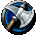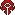This article is a guild progression information page for the Neptulon Europe realm (server). The contents herein are entirely player made and in no way represent official World of Warcraft history or occurrences which are accurate for all realms. This page includes a list of guilds that have accomplished a particular task (usually by defeating a major boss). The list should not be taken to be 100% accurate, as it only contains data that has been submitted/added by users of the community. Thus, if you have information you can add, and contribute to the list, it will become more complete.Hordedon'tremove Karazhan Gruul's Lair Serpentshrine Cavern The Eye Magtheridon Black Temple Mount Hyjal Black Ravens: [URL (w)] x x x x x x x Imbalanced: (w) Complete Complete Complete Complete Complete Complete Complete Isbjörn: [URL (w)] x x x x x x x red Angels: (w) Complete Complete x x x x x The Devastating Throng: [URL (w)] x x x x x x x Wicked: [URL (w)] x x x x x x x VildaBrax: (w) Complete Complete Complete Complete Complete Complete Complete Vildadjoor: [URL (w)] x x x x x x x Unforgiven: [URL (w)] x x x x x x x Underrated: [URL (w)] Complete Complete Hydross the Unstable x Complete x x XW: [URL (w)] Complete x x x x x x Flare: (w) Complete Complete Leotheras the Blind Void Reaver Complete x xAlliance Karazhan Gruul's Lair Serpentshrine Cavern The Eye Magtheridon Black Temple Mount Hyjal Apathy: (w) Complete Complete Complete Complete Complete Complete Complete BG: [URL (w)] Complete Complete Complete Complete Complete Complete Complete Big Bad Mojos: (w) Complete Complete Complete Complete Complete Complete Complete Scorched: (w) x x x x x x x Disbanded: (w) Complete Complete Complete Complete Complete Complete Complete Furious: [URL (w)] Complete Complete Complete Complete Complete Complete Complete Held Hostile: (w) Complete Complete Complete Complete Complete Complete Complete Hellfrost: [URL (w)] x x x x x x x Heroic: [URL (w)] Complete Complete Complete Complete Complete Complete Complete Hypnotic: [URL (w)] x x x x x x x Livonia: (w) Complete Complete Complete 3 Complete 2 4 Magnus: (w) x x x x x x x Raging knights: (w) x x x x x x x Redemption: [URL (w)] Complete x x x x x x Rise Against: (w) Complete Complete Complete Complete Complete 3 4 Screams: [URL (w)] x x x x x x x SIN: [URL http://www.sin-wow.com] Complete Complete Complete Complete Complete x x The Dark Embrace: (w) Complete Complete Complete Complete Complete Complete Complete Volcano Legion: (w) Complete Complete Complete Kael'Thas left Complete x x You No Take Candle: [URL (w)] x x x x x x x Zerg: [URL (w)] x x x x x x x